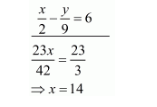# Solve the following systems of equations:

Question:

Solve the following systems of equations:

$\frac{x}{7}+\frac{y}{3}=5$

$\frac{x}{2}-\frac{y}{9}=6$

Solution:

The given equations are:

$\frac{x}{7}+\frac{y}{3}=5 \ldots$(i)

$\frac{x}{2}-\frac{y}{9}=6 \ldots$(ii)

Multiply equation (i) by $\frac{1}{3}$ and add both equations we get

$\frac{x}{21}+\frac{y}{9}=\frac{5}{3}$Put the value of $x$ in equation $(i)$ we get

$\frac{14}{7}+\frac{y}{3}=5$

$\Rightarrow \frac{y}{3}=3$

$\Rightarrow y=9$

Hence the value of $x=14$ and $y=9$.